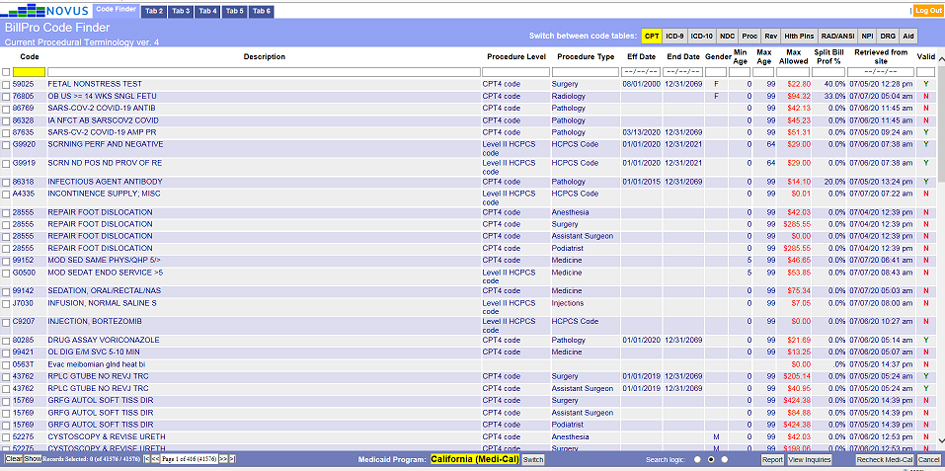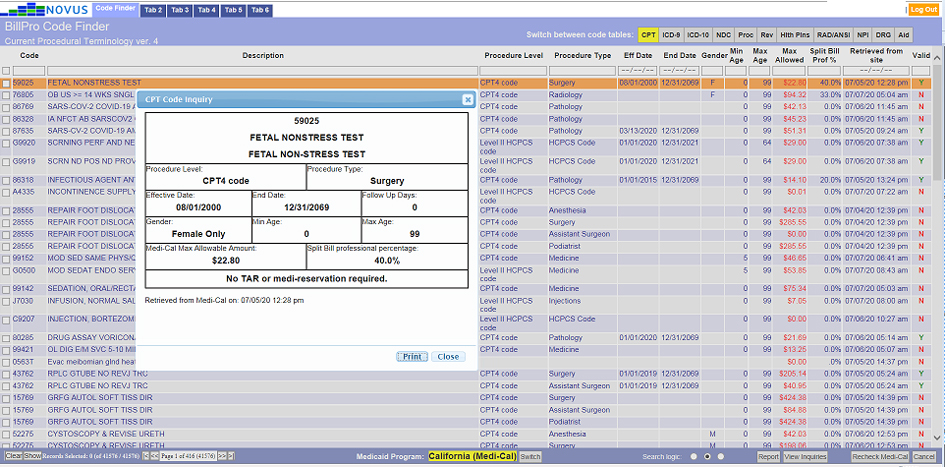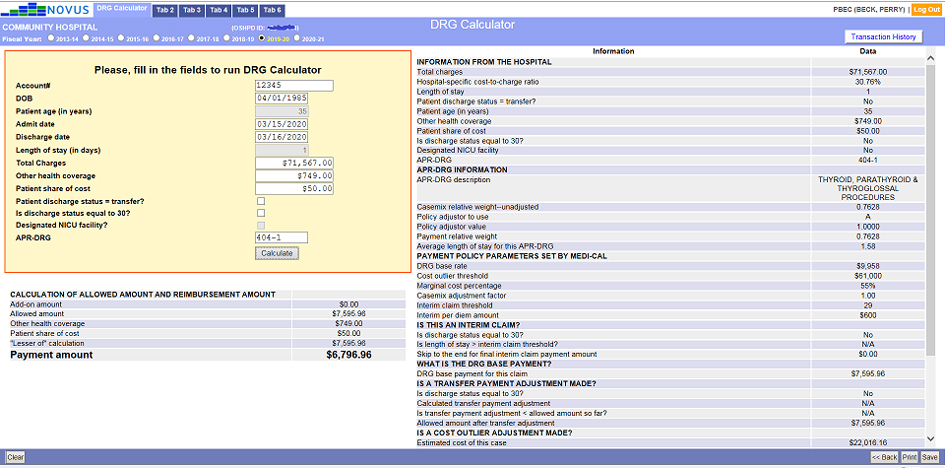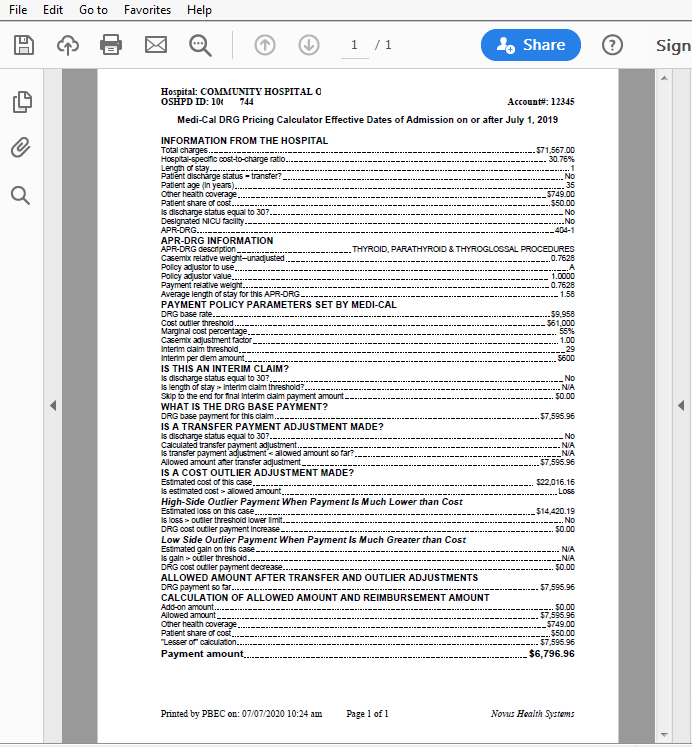## Code Search & Data Sheets

This is a summary of the data populated.This is a summary of the data populated.This is a summary of the data populated.This is a summary of the data populated.This is a summary of the data populated.This is a summary of the data populated.This is a summary of the data populated.This is a summary of the data populated.This is a summary of the data populated.

• In Figure 1 below, the quick links are on the right side• In Figure 2 below, the code finder search area• In Figure 3 below, close up of the specific inquiry• In Figure 4 below, shows one of our several calculators• In Figure 5 below, the quick links are on the right side